﻿ 社会经济与环境污染时空演变及动力机制分析——以重庆市为例

# 社会经济与环境污染时空演变及动力机制分析——以重庆市为例Spatio-Temporal Evolution and Dynamic Mechanism Analysis of Social Economy and Environmental Pollution—Taking Chongqing as an Example

Abstract: Based on the data of county-level scales in Chongqing from 2007 to 2016, this paper explains the dynamic evolution and spatial connection between the socio-economic center of gravity and the center of environmental pollution from multiple perspectives. This paper further analyzes the spatio-temporal evolution characteristics and dynamic mechanism of social economy and envi-ronmental pollution through the spatial interpolation method. Chongqing’s social economic and environmental pollution gravity centers are located in the southwest of the geometric center of gravity, showing an uneven distribution. In addition to the migration of the population’s gravity centers, the distribution of other elements gradually equalizes. The changes in SO2 emissions and GDP gravity centers are more dramatic, and the population gravity centers are relatively stable. The overall difference in GDP and industrial output distribution has narrowed, and the environmental conditions have generally improved, but some pollution centers still exist.

1. 引言

2. 研究方法及数据来源

2.1. 研究区概况

2.2. 研究方法

$X=\frac{{\sum }_{i=1}^{n}{h}_{i}{x}_{i}}{{\sum }_{i=1}^{n}{h}_{i}},Y=\frac{{\sum }_{i=1}^{n}{h}_{i}{y}_{i}}{{\sum }_{i=1}^{n}{h}_{i}}$ (1)

${\theta }_{\left(k+1\right)-k}=\frac{n\text{π}}{2}+arctg\left(\frac{{y}_{k+1}-{y}_{k}}{{x}_{k+1}-{x}_{k}}\right)$ (2)

${D}_{\left(k+1\right)-k}=C×\sqrt{{\left({x}_{k+1}-{x}_{k}\right)}^{2}+{\left({y}_{k+1}-{y}_{k}\right)}^{2}}$ (3)

${V}_{ab}=\frac{{D}_{ab}}{b-a}$ (5)

2.3. 数据来源

3. 重庆市社会经济与环境污染重心演变路径分析

3.1. 人口重心演变轨迹Table 1. The moving direction and distance of social and economical gravity centers in Chongqing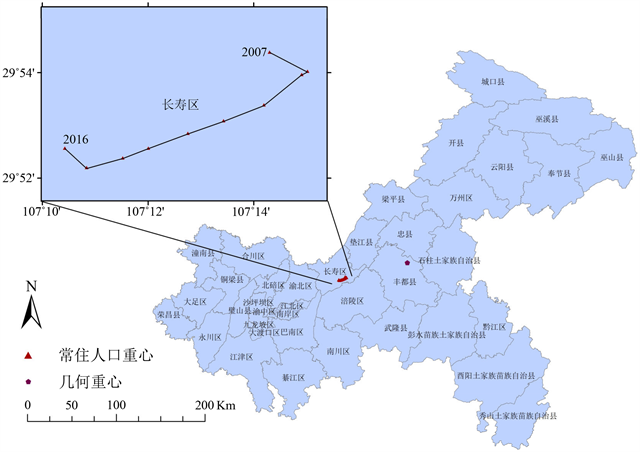(a)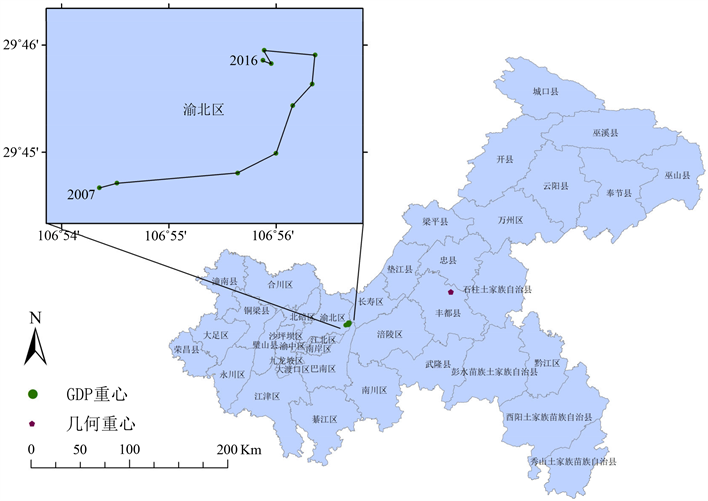(b)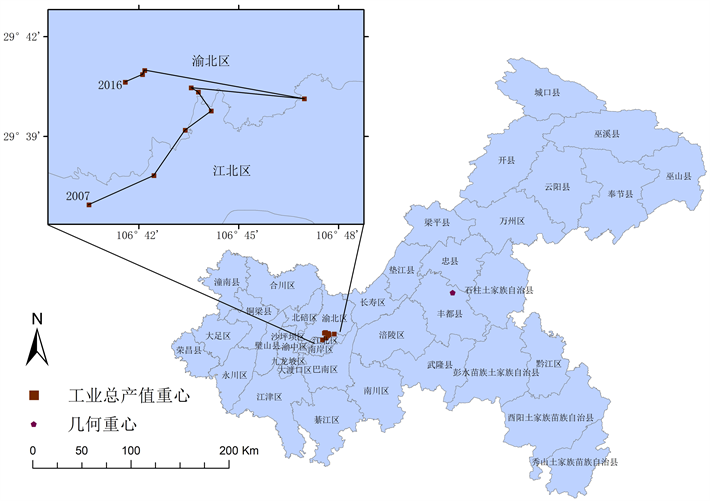(c)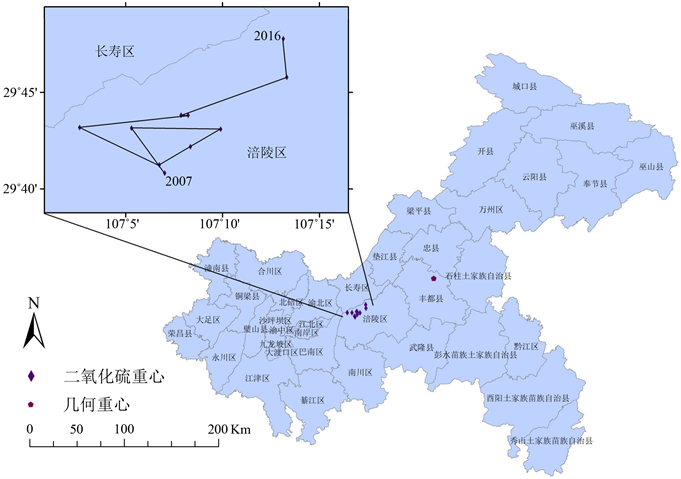(d)

Figure 1. The migration track of the permanent population (a), GDP gravity (b), industrial output gravity (c) and SO2 emissions gravity centers (d)

3.2. GDP重心演变轨迹

3.3. 工业总产值演变轨迹

3.4. 环境污染演变轨迹

4. 迁移机制分析

4.1. 社会经济与环境污染重心演变特征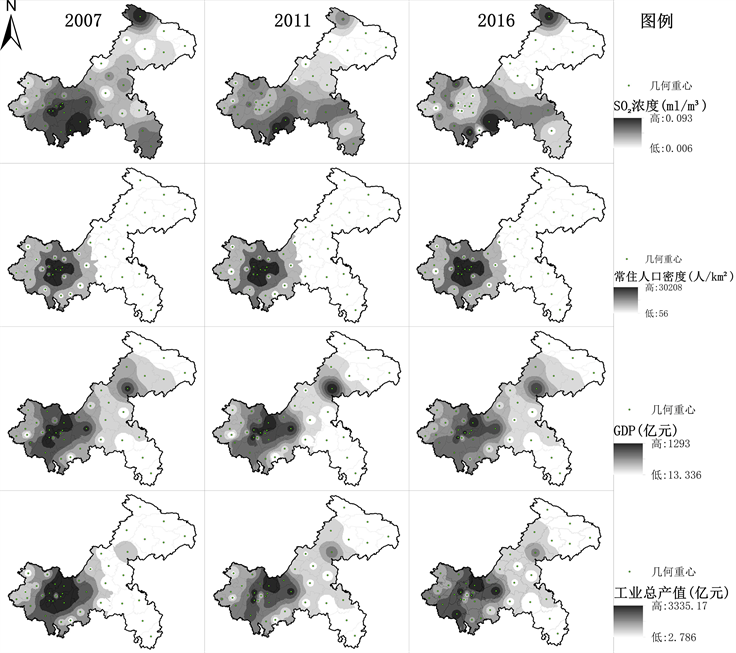Figure 2. Spatial distribution of social economy and environmental pollution in Chongqing in 2007, 2011 and 2016

4.2. 社会经济与环境污染重心迁移对比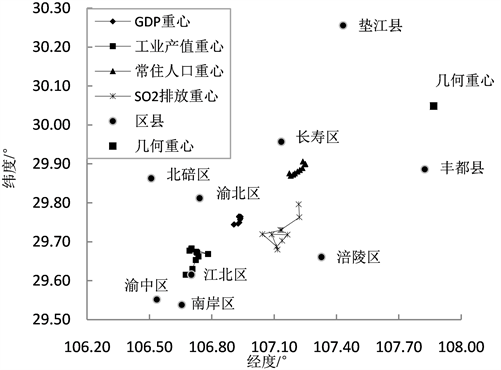Figure 3. Changes in the position of social economy and environmental pollution gravity centers in Chongqing

5. 结论

1) 从地理位置来看，重庆市人口重心、GDP重心、工业总产值重心、SO2排放重心均位于几何重心西南部，呈现沿东北–西南方向分布的基本特征，反映了重庆市人口、社会经济、环境状况分布的不均衡性。

2) 均衡性表现为偏移几何重心距离按以下顺序逐步降低：人口重心、SO2排放重心、GDP重心、工业产值重心，除人口重心向西南方向移动外，GDP重心、工业总产值重心、SO2排放重心均整体向靠近几何重心的东北方向移动，分布趋于均衡化，重庆市社会经济增长与环境污染之间存在着较强的空间联系，与重庆市发展战略密切相关。

3) 在变化的剧烈程度上，人口重心 < 工业产值重心 < GDP重心 < SO2排放重心，SO2排放重心最为活跃，迁移速度最大，人口重心迁移速度最小，位置较为稳定。

4) 基于空间差值法的各指标分布图来看，除人口密度分布变化不大以外，GDP与工业产值较高值区向渝东北、东南扩展，分布整体差异缩小；渝中与渝两翼SO2排放量中低值区域扩大，环境状况整体改善，但仍存在几个高污染中心需要引起重视。

 曹晓仪, 林天应, 张艳芳, 等. 1999-2010年重庆市人口与经济重心迁移研究[J]. 重庆师范大学学报(自然科学版), 2012, 29(1): 43-48.

 李仪俊. 我国的人口重心及其移动轨迹[J]. 人口研究, 1983, 7(1): 28-32.

 乔家君, 李小建. 近50年来中国经济重心移动路径分析[J]. 地域研究与开发, 2005, 24(1): 12-16.

 乔谷阳, 潘少奇, 乔家君. 环境污染重心与社会经济重心的演变对比分析——以河南省为例[J]. 地域研究与开发, 2017, 36(5): 23-28.

 冯宗宪, 黄建山. 1978-2003年中国经济重心与产业重心的动态轨迹及其对比研究[J]. 经济地理, 2006, 26(2): 249-254.

 王欣, 吴殿廷, 肖敏. 产业发展与中国经济重心迁移[J]. 经济地理, 2006, 26(6): 978-981.

 黄建山, 冯宗宪. 我国产业经济重心演变路径及其影响因素分析[J]. 地理与地理信息科学, 2005, 21(5): 49-54.

 涂建军, 刘莉, 张跃, 等. 1996-2015年我国经济重心的时空演变轨迹——基于291个地级市数据[J]. 经济地理, 2018.

Top